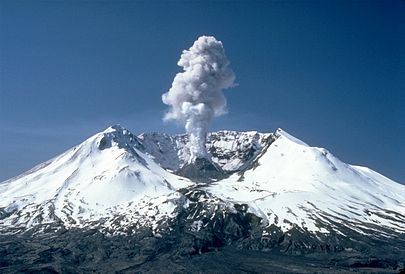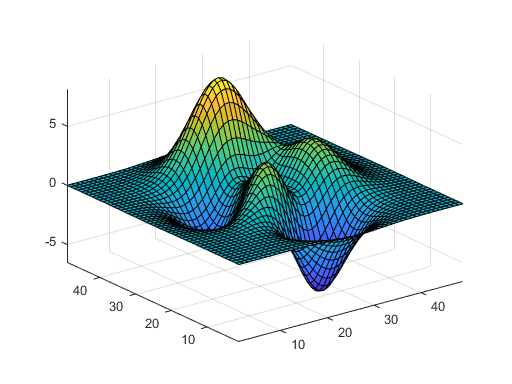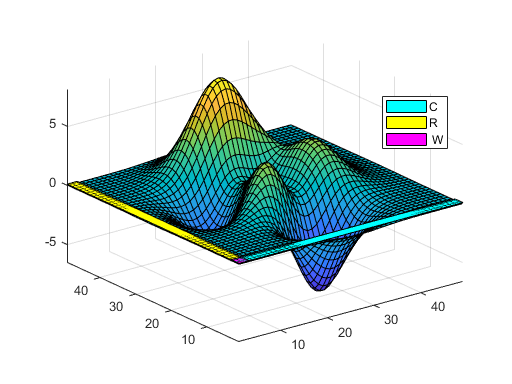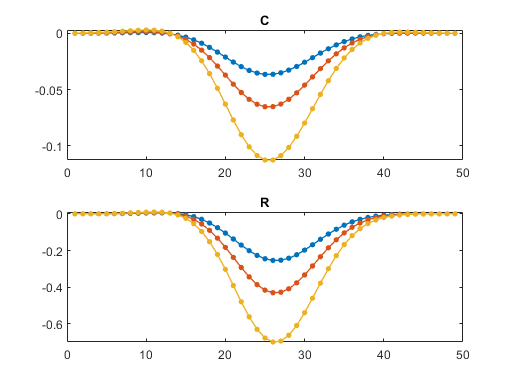# Mount St. Helens and Matrix Rank

What do Mount St. Helens and the rank of a matrix have in common? The answer is the MATLAB function peaks. Let me explain. Please bear with me -- it's a long story.

### Contents

#### Mount St. HelensMount St. Helens is an active volcano in the Cascade Range near Portland, Oregon and Seattle, Washington. She had a dramatic eruption in May 1980. Four years after the eruption, I moved to Beaverton, Oregon to join a spinoff from Intel that was developing one of the world's first commercial parallel computers, the iPSC. In Beaverton, I met two guys from Tektronix, a company famous for oscilloscopes that was then trying to get into computer graphics. These guys, whose names I don't remember, were plotting a surface that resembled Mount St Helens. That surface eventually became the MATLAB function peaks, which is a function of two variables that is useful for demonstrating surf, mesh, pcolor and contour.

   small_fig
clear
P = peaks;
surf(P)
axis tight#### Rank Revealing Factorizations

Two months ago, I wrote a blog post about rank revealing matrix factorizations, It is hard to think of the array P that we obtain from peaks as the representation of a linear transformation and a test matrix, but it is. In fact, for any positive integer n, peaks(n) is an n -by- n matrix. The default has n = 49.

I was very surprised when I discovered that for any n > 2, the rank of peaks(n) is only 3.

   rankP = rank(P)

rankP =

3



#### C*inv(W)*R

MATLAB's rank counts nonzero singular values, but we can also try the factorization P = C*inv(W)*R. We intend to use car only on small matrices that have integer entries. This P is neither small nor integer, but that doesn't stop us. car also finds the rank is 3.

   [C,W,R] = car(P);
whos

  Name        Size            Bytes  Class     Attributes

C          49x3              1176  double
P          49x49            19208  double
R           3x49             1176  double
W           3x3                72  double
rankP       1x1                 8  double



The 49-by-3 matrix C is the first three columns of P and the 3-by-49 matrix R is the first three rows of P. The 3-by-3 matrix W is their intersection, P(1:3,1:3). These three matrices lie safely out of the reach of volcanic eruptions. Here they are, in color.

   d = .1;
n = 49;
r = 3;
s = [patch([1-d n+d n+d 1-d 1-d],[1-d 1-d r+d r+d 1-d],[d d d d d],'c')
patch([1-d r+d r+d 1-d 1-d],[1-d 1-d n+d n+d 1-d],[d d d d d],'y')
patch([1-d r+d r+d 1-d 1-d],[1-d 1-d r+d r+d 1-d],[d d d d d],'m')];
legend(s,{'C','R',' W'}, 'position',[.75 .62 .12 .12]);Plots of C and R do not easily reveal the fact that they contain enough information to reconstruct the entire surface P.

   subplot(2,1,1)
plot(C,'.-','linewidth',1,'markersize',12)
title('C')

subplot(2,1,2)
plot(R','.-','linewidth',1,'markersize',12)
title('R')#### peaks

The function peaks begins by generating matrices x and y that sample a continuous real variable on the interval [-3,3]. Setting the meshsize dx = 1/8 leads to n = 49. Smaller mesh sizes lead to larger matrices, but they all have rank 3.

   dx = 1/8;
[x,y] = meshgrid(-3:dx:3);


The core of peaks is this formula, which must be something like the one that the Tektronix guys were using for their Mt. St. Helens It is not obvious that the formula always generates a matrix of low rank.

   z =  3*(1-x).^2.*exp(-(x.^2) - (y+1).^2) ...
- 10*(x/5 - x.^3 - y.^5).*exp(-x.^2-y.^2) ...
- 1/3*exp(-(x+1).^2 - y.^2);


#### Rank

The rest of this post is devoted to proving that rank(z) = 3.

Here are two important facts about the rank of sums and pointwise products.

• rank(A + B) <= rank(A) + rank(B)
• rank(A.*B) <= min(rank(A),rank(B))

In particular, if A and B are both rank one, then

• rank(A + B) <= 2
• rank(A.*B) <= 1

#### Reformulate

Let's factor out the exponentials using this function.

   E = @(x,a) exp(-(x+a).^2);


So, here is z again.

   z =  3*(1-x).^2.*E(x,0).*E(y,1) ...
- 10*(x/5 - x.^3 - y.^5).*E(x,0).*E(y,0) ...
- 1/3.*E(x,1).*E(y,0);


Let z1 be the first term.

   z1 =  (3*(1-x).^2).*E(x,0).*E(y,1);


rank(z1) = 1 because z1 is the pointwise product of three matrices. There is no sum.

Let

   zx = -10*(x/5 - x.^3).*E(x,0) - 1/3.*E(x,1);
zy = (10*y.^5).*E(x,0);


rank(zx) = 1 because zx does not involve y.

rank(zy) = 1 because zy is a pointwise product.

   z2 =  (zx + zy).*E(y,0);


rank(z2) = (1 + 1)*1 = 2

   z = z1 + z2;


rank(z) = 1 + 2 = 3

#### Check

My original P is still in the workspace.

  check = norm(P - z,inf)

check =

1.3517e-14



Published with MATLAB® R2020a

|

### Comments

To leave a comment, please click here to sign in to your MathWorks Account or create a new one.